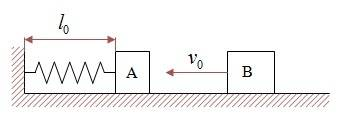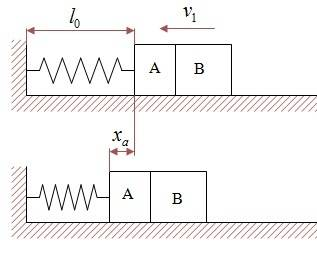# 2 masses, friction and a spring

• Karol
In summary, the problem involves two boxes, A and B, with different initial velocities. They collide plastically without clinging to each other and the spring they collide against is initially loose. The collision time is assumed to be very short. The problem asks for the velocity of box B immediately after the collision, the distance from the wall where box A initially stops, the force resultant on box A immediately after it stops, the distance from the wall where box B detaches from A, and the final stopping point of box B. Additionally, box A continues to oscillate until it stops at a certain point xf, and the problem also asks for the points x1 and x2 at which A has momentary maximum velocity, and whether xf is greater

## Homework StatementBox B has initial velocity v0 and collides plastically but without clinging to mass A which is at rest.
The spring, initially, is loose. assume that the collision time is very short.
What's the boxes's velocity immediately after the collision?
At what distance from the wall box A stops initially?
What's the force resultant on box A immediately after it's stop at the first time?
At what distance from the wall will box B detach from A?
Where will B finally stop?
A continues to oscillate until it stops at xf. find points x1 and x2 at which A has momentary maximum. will xf be:
xf>x2, x1≤xf≤x2, xf<x1
The problem states quantities for everything: masses etc. but i don't give them, i solve in principle.

## Homework Equations

Kinetic energy: ##E=\frac{1}{2}mv^2##
Potential energy: ##E=\frac{1}{2}kx^2##
Conservation of momentum: ##m_1v_1+m_2v_2=m_1v_1'+m_2v_2'##

## The Attempt at a Solution[/B]
Conservation of momentum:
$$m_Bv_0=(m_A+m_B)v_1\;\rightarrow\; v_1=\frac{m_B}{m_A+m_B}v_0$$
The kinetic energy is transformed into potential+friction loss:
$$\frac{1}{2}(m_A+m_B)v_1^2=(m_A+m_B)\mu g+\frac{1}{2}kx_a^2\;\rightarrow\;x_a=...$$
Immediately after both stop for the first time, the force on A is ##F=kx_a-(m_A+m_B)\mu g##
B will detach from A at the relaxed length of the spring, at x=l0, since even though the force the spring applies, kx, is smaller than the friction of any or both together, at some point, it is still acting on both masses. in order for B to detach A must be drawn backwards, it's not enough that there will be 0 net force on it.
I feel this isn't right but i have no other solution.
The potential energy when both stop at xa is transformed into kinetic energy at x=0 plus friction loss:
$$\frac{1}{2}kx_a^2=\frac{1}{2}(m_A+m_B)v_3^2+(m_A+m_B)\mu g x_a\;\rightarrow\;v_3=...$$
The points on both sides of x=0: ##kx=m_Ag\mu\;\rightarrow\; x=\frac{m_Ag\mu}{k}## are the ones with temporary maximum velocity.
mA won't stop outside x1 or x2 since if the mass stops, while oscillating, temporarily there, the spring's force will overcome friction.
If mA stops temporarily just outside one of these x'ses, the friction loss, in order to reach the other x, is greater than the potential energy:
$$\frac{1}{2}kx_a^2-2\cdot m_Ag\mu x_a=...<0$$
So mA will finally halt between them.

Last edited:
Hi,

I see a problem in your second equation. All terms must be energies, but one is not.

$$\frac{1}{2}(m_A+m_B)v_1^2=(m_A+m_B)\mu g x_a+\frac{1}{2}kx_a^2\;\rightarrow\;x_a=...$$

Karol said:
Immediately after both stop for the first time, the force on A is ##F=kx_a-(m_A+m_B)\mu g##
What about the normal force from B on A? Also why should the weight of B affect the frictional force on A?
(Rather than consider the individual forces on A, I would calculate the acceleration of the boxes and then use FA = mAa)

As for how to find the point at which B loses contact with A:
If B clung to A, then at some point the normal force would vanish and then begin to become negative. This moment of vanishing normal force is when B will lose contact with A.

$$F_{tot}=kx_a-(m_A+m_B)\mu g=(m_A+m_B)a\;\rightarrow\; a=\frac{kx_a-(m_A+m_B)\mu g}{m_A+m_B}$$
$$F_A=m_A\cdot a\;\rightarrow\; F_A=\frac{m_A}{m_A+m_B}(kx_a-(m_A+m_B)\mu g)$$
A and B will start to decelerate at ##x=\frac{(m_A+m_B)\mu g}{k}##. there the normal force between A and B will vanish, but that doesn't seem good to me.

Karol said:
A and B will start to decelerate at ##x=\frac{(m_A+m_B)\mu g}{k}##. there the normal force between A and B will vanish, but that doesn't seem good to me.
You are just assuming that the normal force will vanish when they begin to decelerate? If there was no friction, I would agree. But, because there is friction, it is possible for the blocks to decelerate with the normal force still being positive.

The systematic approach would be to consider the net force on each box. The boxes have the same acceleration (at least until after they lose contact) so we can say Fnet.A/mA = Fnet.B/mB ... ←that equation will involve the normal force FN and the compression x. If you let FN vanish then you will see what x must be.

I will just say the result because I am about to sleep: Your first guess was actually correct, it will occur at the rest length of the spring (when x=0). The reason I make you go through this "vanishing normal force" approach is because you were unsure about how (and I did not understand how) you got that result. The general approach (in many types of problems) for finding when two objects lose contact is to find where the normal force between them vanishes (and would become negative if they were "clung" together).

I have not checked your work on the rest of the problem (sorry) but I will tomorrow if no one else has taken over by then.

Nathanael said:
it will occur at the rest length of the spring (when x=0).
The easy way to see this is to consider that they will separate when A's acceleration drops below B's. Since B's will be -μg then, A's must be a bit more negative than due to friction alone. Hence the spring must be starting to pull the other way.

•Nathanael
$$\frac{F_A}{m_A}=\frac{F_B}{m_B} \;\rightarrow\; \frac{m_A g\mu-F_N+kx}{m_A}=\frac{m_B g\mu+F_N}{m_B}$$
$$F_N=0\;\rightarrow\; x=0$$
About the force on A immediately after first stop i guess what i did i post #5 is correct, right?

Karol said:
$$\frac{F_A}{m_A}=\frac{F_B}{m_B} \;\rightarrow\; \frac{m_A g\mu-F_N+kx}{m_A}=\frac{m_B g\mu+F_N}{m_B}$$
$$F_N=0\;\rightarrow\; x=0$$
About the force on A immediately after first stop i guess what i did i post #5 is correct, right?
Yes, it is correct. Also your work on the other parts all looks correct, except that you didn't finish the "what distance will B stop from the wall" part (you found "V3," but you still must say how far that V3 will take the block).

The distance of B from the wall is simple: kinetic energy transferred to friction loss by ##m_B\mu g x##
I guess it's correct, so thank you nathanael also